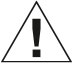# Kids First Math: Linking Cubes Math Kit with Activity Cards

\$18.95
SKU: 568001
##### Product Description

Time after time, experience shows that learning by manipulating physical objects with one’s own hands — learning by doing things firsthand, rather than being told about them or reading about them in a book — is extremely effective. Thames & Kosmos has a long history of incorporating this principle into science education. Now, we are bringing this experience to the Kids First Math line, a series of nine hands-on kits with activity cards or lesson guides designed to demonstrate and teach concepts in mathematics, as well as other skills such as logical thinking, problem solving, and creative thinking.

Kids First Math: Linking Cubes Math Kit with Activity Cards

+ A set of 100 colorful plastic cubes that connect together

+ Used to develop skills in counting, sorting, sequencing, graphing, pattern recognition, measuring, and basic mathematical operations

+ Also develops skills in spatial intelligence, creativity, problem solving, and fine motor skills

+ Cubes can connect on all six sides and rotate at each connection point.

+ Endless possibilities for open-ended creative play and building

+ Durable plastic for long-term use and easy cleaning

+ Includes 100 plastic connecting 2-cm cubes in 10 colors, 5 activity cards, and plastic storage boxWARNING: CHOKING HAZARD — Small parts. Not for children under 3 yrs.
##### Product Information

Ages: 3+
Experiments: 10
Piece Count: 105
Manual Pages: 10
Product Dimensions: 7.5 x 2.8 x 5.9 in.
Product Weight: 1.3 lbs
Manual Dimensions: 5.8 x 4.1 in.
Batteries Required: N/A
Country of Origin: Taiwan
Year Released: 2021

##### CCSS Correlations

K.CC.A.1 Know number names and the count sequence.

K.CC.A.2 Know number names and the count sequence.

K.CC.A.3 Know number names and the count sequence.

K.CC.B.4 Count to Tell the Number of Objects

K.CC.B.5 Count to Tell the Numbers of Objects

K.CC.C.6 Compare Numbers

K.OA.A.1 Understand addition as putting together and adding to, and understand subtraction as taking apart and taking from.

K.OA.A.2 Understand addition as putting together and adding to, and understand subtraction as taking apart and taking from.

K.OA.A.3 Understand addition as putting together and adding to, and understand subtraction as taking apart and taking from.

K.OA.A.4 Understand addition as putting together and adding to, and understand subtraction as taking apart and taking from.

K.OA.A.5 Understand addition as putting together and adding to, and understand subtraction as taking apart and taking from.

K.NBT.A.1 Work with numbers 11-19 to gain foundations for place value.

K.MD.A.1 Describe and Compare Measurable Attributes

K.MD.A.2 Describe and Compare Measurable Attributes

K.MD.B.3 Classify objects and count the number of objects in each category

K.G.A.4 Analyze, Compare, Create, and Compose Shapes

K.G.A.6 Analyze, Compare, Create, and Compose Shapes

1.OA.A.1 Represent and solve problems involving addition and subtraction.

1.OA.A.2 Represent and solve problems involving addition and subtraction.

1.OA.B.3 Understand and apply properties of operations and the relationship between addition and subtraction.

1.OA.B.4 Understand and apply properties of operations and the relationship between addition and subtraction.

1.OA.C.5 Add and subtract within 20.

1.OA.C.6 Add and subtract within 20.

1.OA.D.7 Work with addition and subtraction equations.

1.OA.D.8 Work with addition and subtraction equations.

1.MD.A.1 Measure lengths indirectly and by iterating length units.

1.MD.A.2 Measure lengths indirectly and by iterating length units.